# Conjugacy class size formula in general linear group over a finite field

(diff) ← Older revision | Latest revision (diff) | Newer revision → (diff)
Jump to: navigation, search

This article gives formula(s) for the conjugacy class sizes in a general linear group of finite degree$n$ over a finite field with$q$ elements, which we denote by$GL(n,q)$.

## Case of semisimple elements

### Elements diagonalizable over$\mathbb{F}_q$

Suppose$g \in GL(n,q)$ is diagonalizable over$\mathbb{F}_q$, with eigenvalues$\lambda_1, \dots, \lambda_k$ having multiplicities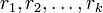$r_1,r_2,\dots,r_k$ respectively (the$\lambda_i$s are all distinct). Note that$\sum_{i=1}^k r_i = n$.

Then, the centralizer of the diagonal representative of this conjugacy class is isomorphic to:$GL(r_1,q) \times GL(r_2,q) \times GL(r_3,q) \times \dots \times GL(r_k,q)$

In fact, if the diagonal entries are arranged so that all the$\lambda_1$s occur first, then the$\lambda_2$s, and so on, then the centralizer is the set of invertible block diagonal matrices with blocks of sizes$r_1, r_2, \dots, r_k$.

The size of the conjugacy class is thus:$\frac{|GL(n,q)|}{\prod_{i=1}^k |GL(r_i,q)|}$

This is the same as the$q$-analogue of the multinomial coefficient:$\binom{n}{r_1,r_2,\dots,r_k}_q$

In all cases, this simplifies to a polynomial in$q$, and its degree is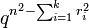$q^{n^2 - \sum_{i=1}^k r_i^2}$.

Some particular cases for the partition of$n$ as a sum of$r_i$s, and the corresponding sizes, are given below.$n$ Partition of$n$ Size of$|GL(n,q)|$ Size of centralizer of diagonal element Degree of polynomial giving size Size of conjugacy class Degree of polynomial giving size
1 1$q - 1$$q - 1$ 1 1 0
2 2$(q^2 - 1)(q^2 - q)$$(q^2 - 1)(q^2 - q)$ 4 1 0
2 1 + 1$(q^2 - 1)(q^2 - q)$$(q - 1)^2$ 2$q(q + 1)$ 2
3 3$(q^3 - 1)(q^3 - q)(q^3 - q^2)$$(q^3 - 1)(q^3 - q)(q^3 - q^2)$ 9 1 0
3 2 + 1$(q^3 - 1)(q^3 - q)(q^3 - q^2)$$(q^2 - 1)(q^2 - q)(q - 1)$ 5$q^2(q^2 + q + 1)$ 4
3 1 + 1 + 1$(q^3 - 1)(q^3 - q)(q^3 - q^2)$$(q - 1)^3$ 3$q^3(q^2 + q + 1)(q + 1)$ 6$n$$n$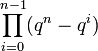$\prod_{i=0}^{n-1} (q^n - q^i)$$\prod_{i=0}^{n-1} (q^n - q^i)$$n^2$ 1 0$n$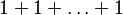$1 + 1 + \dots + 1$$\prod_{i=0}^{n-1} (q^n - q^i)$$(q - 1)^n$$n$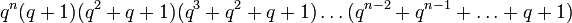$q^n(q + 1)(q^2 + q + 1)(q^3 + q^2 + q + 1) \dots (q^{n-2} + q^{n-1} + \dots + q + 1)$$n(n - 1)$